• Call Now

1800-102-2727•

# Difference between Ammeter and Voltmeter-Practice problems, FAQs

Sachin buys three bulbs from the hardware store. Let’s name these bulbs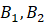and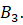He connects them in parallel to an AC source using wires and finds that the bulb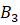fuses after some time. He is confused why this happens; so he does a little research on the internet and learns that any electrical appliance can only withstand a certain amount of current. For instance, let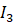indicate the safe current that can be passed through bulb. Let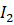be the safe current that can be passed through bulb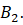It is clear thatis greater than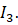But how to know their values before allowing them to pass through the bulb? A device called ammeter is going to help Sachin know this. Similar to the above, each electrical equipment has a safe voltage that it can withstand before it fuses. For instance, a bulb rated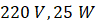means that it can safely operate on a source voltage up to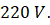How to know this value? A device called voltmeter is needed for this. In this article, we will explore ammeter and voltmeter in detail.

## What is an ammeter?

Ammeter is a device used for measuring currents. On the other hand, a galvanometer is used to measure smaller currents. An ammeter is always connected in series in a circuit. It has a needle which deflects on a graduated scale. The scale is graduated in amperes (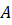) or milliamperes (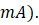An ideal ammeter is one which has zero resistance.

Let us consider the following circuit where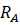indicates the resistance of the ammeter and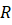indicates the external resistance. The current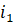flowing in the circuit can be written as,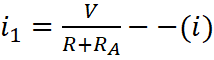.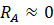by definition of an ideal ammeter.

Then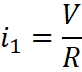I.e. the reading would be the same as when a resistoris connected. From equation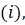it is clear that lower the resistance of the ammeter, more accurate will the reading be.

## What is a voltmeter?

A voltmeter is a device used for measuring potential difference across an appliance. A voltmeter is always connected in parallel in a circuit. It has a needle which deflects on a graduated scale. The scale is graduated in volts (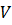) or millivolts (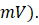An ideal voltmeter is one which has infinite resistance. Higher the resistance of the voltmeter, more accurate would be its reading.

## Conversion of galvanometer to an ammeter

A galvanometer can be converted into an ammeter by connecting a low resistance called shunt in parallel with it; so that the majority of the current will be allowed to pass through the shunt while only a small portion of the current will pass through the galvanometer

Letbe the current in the circuit and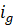be the full scale deflection of the galvanometer.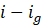is the current that will pass through the shunt. The combination of galvanometer and shunt will behave as an ammeter. Let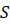be the value of shunt resistance and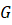indicate the galvanometer resistance. Since they are connected in parallel, their voltage drop would be the same, meaning the product of their respective currents and resistances are equal.

I.e,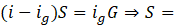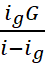The above equation gives the value of shunt resistance which needs to be connected in parallel with the galvanometer to be able to measure current.

The effective resistance of the ammeter so formed is given by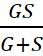.

## Conversion of galvanometer to a voltmeter

A galvanometer is converted into a voltmeter by connecting a high resistance in series with it. In the following diagram, letindicate the resistance of the galvanometer. The resistor to be used in series is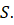Letbe the voltage across the combination, then by Ohm’s law,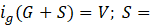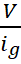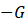The above equation gives an idea about the resistance that needs to be connected in series with the galvanometer to convert into voltmeter.

## Practice problems

Q1. Construct a voltmeter from a galvanometer whose resistance is given by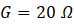and a full scale deflection of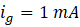into a voltmeter with a maximum range of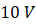Answer: Given , galvanometer resistance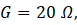,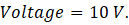Letbe the resistance that needs to be connected in series. Then,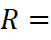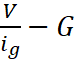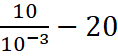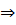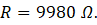Q2. Calculate the value of shunt resistance required to convert a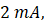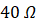galvanometer into an ammeter with a range of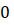to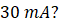(a)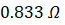(b)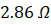( c )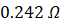(d)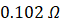Given, full scale deflection of the galvanometer,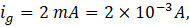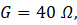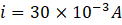The value of shunt resistance that needs to be connected is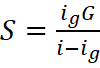Solving, we get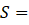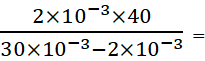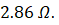Q3. A bulb having a resistance of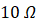is connected to an external resistor having a resistance of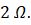The emf of the battery supplying current is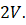Calculate the current flowing in the circuit?

(a)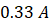(b)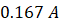( c )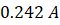(d)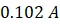Total resistance of the circuit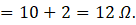Current flowing in circuit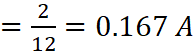Q4. A cell having an emf of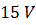supplies a current of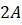to one of the resistor in a circuit having resistance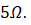A voltmeter is connected in parallel across this resistor. Find its reading?

(a)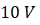(b)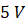( c )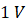(d)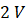Given,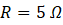and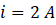By Ohm’s law,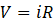.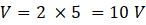## FAQs

Question 1. What is the typical range of an ammeter?
Answer:  An ammeter typically measures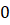to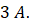It carries about 30 divisions on its scale.

Question 2. What is the least count of a voltmeter?
Answer: The least count or smallest possible measurement that can be made with a voltmeter is generally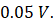Question 3. What is the SI unit for potential difference?
Answer:The SI unit for potential difference is volt.

Question 4. Write one use of a moving coil galvanometer?
Answer: It is used to measure small currents.Talk to our expert
Resend OTP Timer =
By submitting up, I agree to receive all the Whatsapp communication on my registered number and Aakash terms and conditions and privacy policy